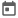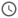# Basics of Verilog##### 12 Aug 2021##### 4 mins

Verilog is based on modular programming, i.e., the designs are written in small modules and then it can be combined to make a complex design. Modules can be thought of as classes, expect the fact that modules are not dynamic, i.e., objects cannot be made of modules as we can do for classes in OOPs language. But modules can be instantiated multiple times and can thus be reused just like classes.
From hardware point of view, every circuit will have a input and output port. Thus, for every module we have to also write the different I/O ports associated with it.

### Verilog Template

Every module in verilog follows a common template. Steps to write Verilog module:

1. First a module is declared using `module` keyword followed by the name of the module. At the end of module statement `;` is a must. Each module statemtent will also have a `endmodule` associated with it, which marks the end of the module.
``````module [module_name];
// code goes here
endmodule``````
1. If module have I/O pins then the ports are defined after the module name using simple brackets `()`.
``````module [module_name]([port_list]);
// code goes here
endmodule``````
1. Sometimes, we may need to use some internal variables, i.e., variables which are not directly accessed from outside the modules. So we need to declare these internal variables.
2. If we want to use some other module inside our module then we instantiate that module and properly connect with them. The syntax to instantiate a module is:
`[module name] [identifier] ([port-connection])`
3. Now we write the code for this module.

So the final template looks like:

``````module [module_name]([port_list]);
[internal_variables]

[instantiation of a another module if required]

// code related to the module goes here
endmodule``````

#### Example

In this example, we will design a 1-bit half adder. For half adder, there are 2 inputs, in this case, A & B and 2 outputs, sum & carry.

``````// Step-1: Declare the half_adder module
module half_adder(input A, B,
output sum, carry)

// Step-2: Internal variable (optional)
reg [1:0] temp;

// Step-3: As this is simple design thus no other module is required.

// Step-4: Module logic goes below
always @(A or B) begin
temp <= A+B;
end

assign sum = temp;
assign carry = temp;
endmodule``````

### Testbench

To run any code, we need to provide some inputs to the code. As in Verilog we are representing hardware, thus the inputs and outputs will be in the form of signals. To give the input as signals we need to make a test bench, from which we can provide inputs to our design and get the output. In technical terms, the design which is tested is known as Device under Test (DUT) or Device under verification (DUV).

#### Steps to write the testbench:

1. Declare a module for testbench as we do in design. But for testbench module, there is commonly no I/O ports required as we will generate the input for our design in this module.
2. Declare internal variables which will act as the input/output to our design.
3. Instantiate the design module and connect the internal variables with correct I/O ports.
4. Generate different inputs.

#### Example

Let's see how the test bench for the half adder module will be like.

``````// Step-1: Declaring the tesbench module
module tb;

// Step-2: define internal variables for DUT
// In this case as there are 2 i/p and 2 o/p
// thus total 4 variables will be required
reg a, b;       // 2 input variables (any valid name can be used)
wire sum, carry;// 2 output variables (any valid name can be used)

// Step-3: instantiate half_adder module and provide a name
half_adder dut(a, b, sum, carry);

// Step-4: Generate inputs for the dut.
initial begin
\$monitor("Input a=%b, b=%b & Output sum=%b, carry=%b", a, b, sum, carry);

a=0; b=0;

#10 a=0; b=1;
#10 a=1; b=0;
#10 a=1; b=1;
#20 \$finish;
end
endmodule``````
##### Output of the half adder test bench
``````Input a=0, b=0 & Output sum=0, carry=0
Input a=0, b=1 & Output sum=1, carry=0
Input a=1, b=0 & Output sum=1, carry=0
Input a=1, b=1 & Output sum=0, carry=1``````